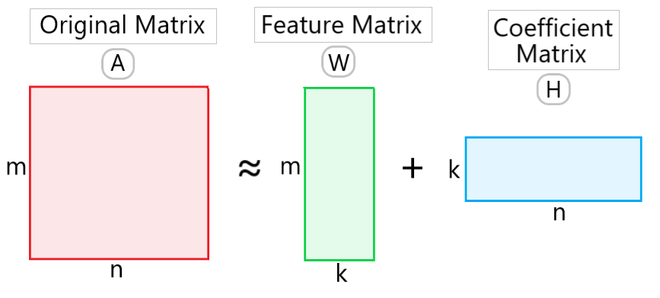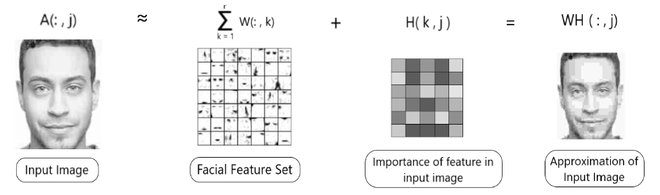# Non-Negative Matrix Factorization

• Last Updated : 22 May, 2022

Prerequisite : Low Rank Approximation

Non-Negative Matrix Factorization: For a matrix A of dimensions m x n, where each element is ≥ 0, NMF can factorize it into two matrices W and H having dimensions m x k and k x n respectively and these two matrices only contain non-negative elements. Here, matrix A is defined as:```where,
A -> Original Input Matrix (Linear combination of W & H)
W -> Feature Matrix
H -> Coefficient Matrix (Weights associated with W)
k -> Low rank approximation of A (k ≤ min(m,n))```

This method is widely used in performing tasks such as feature reduction in Facial Recognition and for various NLP tasks.

Intuition:Fig 1 : NMF Intuition

The objective of NMF is dimensionality reduction and feature extraction. So, when we set lower dimension as k, the goal of NMF is to find two matrices W ∈ Rm×k and H ∈ Rn×k having only nonnegative elements. (As shown in Fig 1)

Therefore, by using NMF we are able to obtain factorized matrices having significantly lower dimensions than those of the product matrix. Intuitively, NMF assumes that the original input is made of a set of hidden features, represented by each column of W matrix and each column in H matrix represents the ‘coordinates of a data point’ in the matrix W. In simple terms, it contains the weights associated with matrix W.

In this, each data point that is represented as a column in A, can be approximated by an additive combination of the non-negative vectors, which are represented as columns in W.

Real-life example:

Let us consider some real-life examples to understand the working of the NMF algorithm. Let’s take a case of image processing.

Suppose, we have an input image, having pixels that form matrix A. Using NMF, we factorize it into two matrices, one containing the facial feature set [Matrix W] and the other containing the importance of each facial feature in the input image, i.e. the weights [Matrix H]. (As shown in Fig 2.)Fig 2 : NMF in Image Processing

NMF is used in major applications such as image processing, text mining, spectral data analysis and many more. Currently, there is an ongoing research on NMF to increase its efficiency and robustness. Other research is being done on collective factorization, efficient update of matrices etc. as well. For any doubt/query, comment below.

My Personal Notes arrow_drop_up